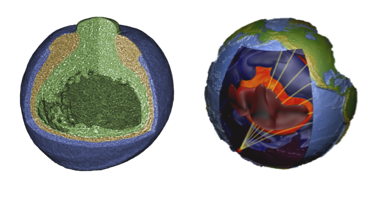### Inverse Problems in Imaging - M1 - 6EC

Aim of the course

This course is about inverse problems in imaging. The mathematical reconstruction and processing of images is of fundamental importance in state of the art applications in health and geosciences, e.g. in medical tomography, in high-resolution microscopy or in geophysical inversion. In many cases, underlying inverse problems can be formulated and solved using variational methods and partial differential equations. This course offers a theoretical as well as an applied insight into inverse problems and variational methods for mathematical imaging. It addresses reconstruction problems of different imaging modalities (e.g. CT or PET) in biomedicine and geophysics. The course covers the full chain of solving inverse problems in imaging, namely

Problem identification → Modeling and discretization → Analysis → Numerical optimization

Where variational principles, regularization theory and numerical optimization (scientific computing) form the underlying joint core. The course connects and extends upon the main concepts of basic courses on differential equations and numerics. The main learning goal for the students is to model, analyze and use state-of-the-art variational methods, PDEs and optimization techniques to solve challenging inverse problems in imaging. Upon completing this course, students achieved the following learning goals:

• Problem identification: Identification of imaging problems as mathematical inverse operator problems (e.g. integral equations, dynamical systems);
• Modeling and discretization: Problem formulation arising in applications using the language of nonlinear variational methods and partial differential equations; use Bayesian modeling to take data and model uncertainty into account; continuous versus discrete modeling;
• Analysis: Understanding the main concepts of nonlinear regularization theory in an analogous way in PDEs as well as in variational methods, and how it influences existence (duality, weak topologies, Theorem of Banach-Alaoglu) and uniqueness results;
• Numerical optimization: Formulating optimality conditions (variations) for constrained convex variational methods (saddle point problems) and to solve them via primal-dual methods or discretized higher-order methods.

At the end of the course participants will be able to tackle inverse problems for imaging in biomedicine or geophysics with a new repertoire of state-of-the-art mathematical tools.

Prerequisites

The course is aimed at Master and starting PhD students in Mathematics (and Technical Medicine) at the comprehensive as well as the technical universities. Solid knowledge of linear algebra and calculus are essential. Furthermore, knowledge of differential equations and numerics is desirable. All at the Bachelor level.

Course material

The lecture notes can be found here.

Lecturers

Tristan van Leeuwen (Mathematics, University of Utrecht) t.vanleeuwen@uu.nl

Christoph Brune (Mathematics, University of Twente) c.brune@utwente.nl